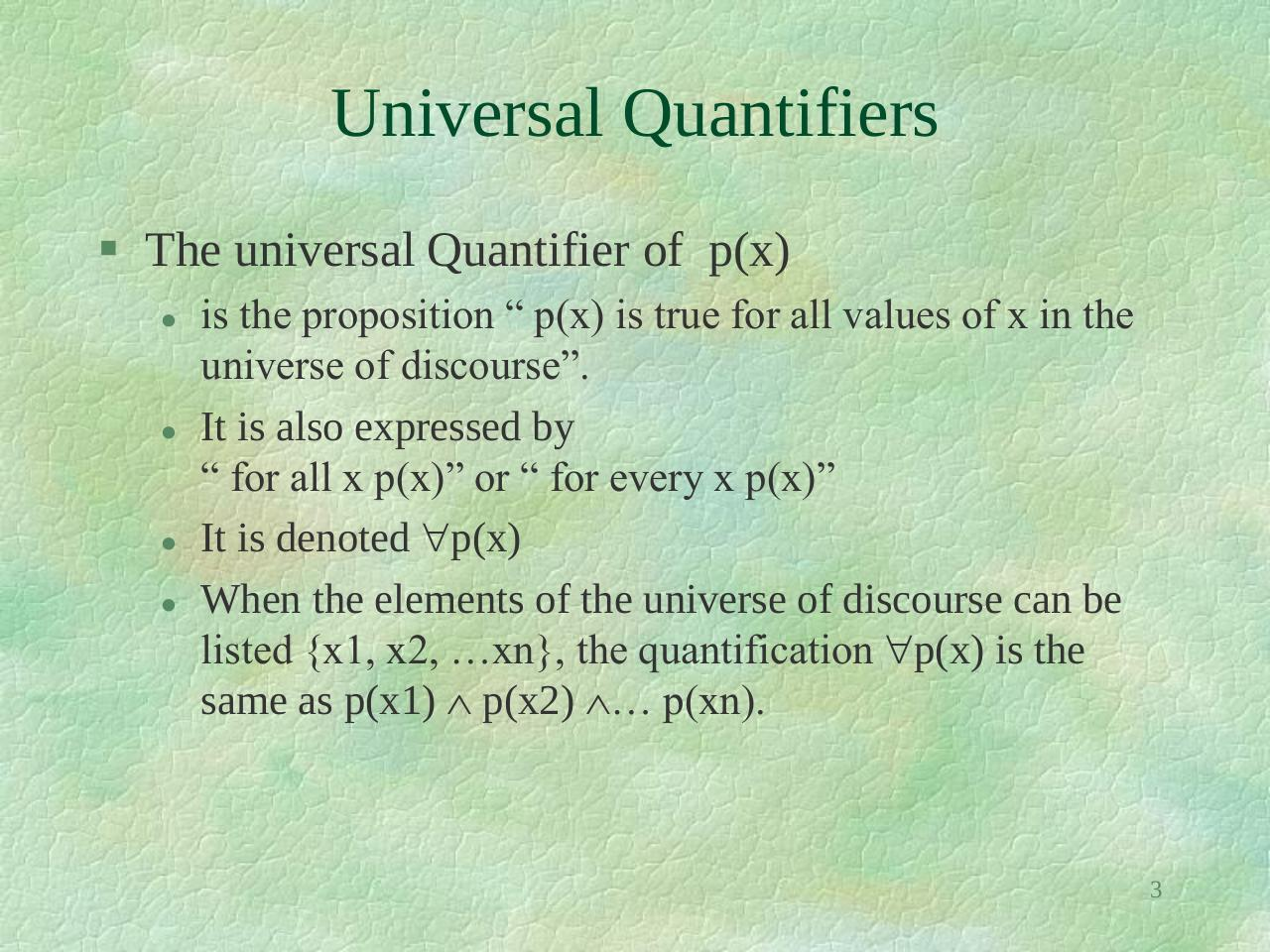Discreet lec 3.pdfPage 1 2 3 4 5 6 7 8 9 10

Text preview

Universal Quantifiers
 The universal Quantifier of p(x)

is the proposition “ p(x) is true for all values of x in the
universe of discourse”.
It is also expressed by
“ for all x p(x)” or “ for every x p(x)”
It is denoted p(x)
When the elements of the universe of discourse can be
listed {x1, x2, …xn}, the quantification p(x) is the
same as p(x1)  p(x2) … p(xn).

3